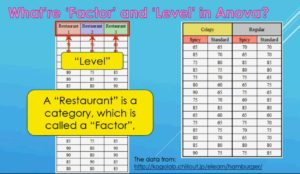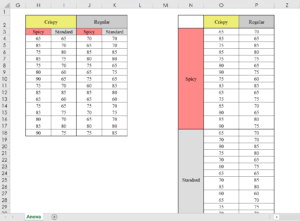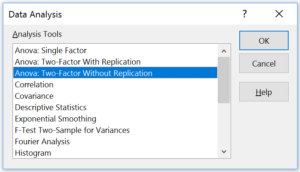Achieve Innovative Process Improvement＋Standardization by IT System with MS 365.# What’s ANOVA? (Analysis of Variance Basics) 【Excel Data Analysis Tools】 Part 1: Get the results quickly\ この記事を共有 /
What's ANOVA? (Analysis of Variance ...

With Anova, you can identify if there is significant difference among the data groups. This article explains the basics of Anova and demonstrate the Two-Factor Anova using Excel’s Data Analysis tools.

(Duration: 6:19)

## Anova Basics and Difference between Anova and T Test

Hi, this is Mike Negami, Lean Sigma, Black Belt.

Today’s topic is from this request:“Since I’ll have an exam, I would like to see videos about Single Factor Anova, Two-Factor Anova!”

By the way, let me give a short note: I try to avoid using complex formulas and jargon in my statistics videos. Instead, I’ll focus on how to use these amazing tools in practice.

Before talking about Single Factor and Two-Factor Anova, let’s review the basics of Anova. Anova is an abbreviation of Analysis of Variance.

With Anova, by comparing and analyzing the means of multiple data groups, you can identify if there is significant difference among the data groups.

For example, you can compare the data before and after your multiple branches run a sales campaign and examine the campaign’s effects or compare the performance of multiple production lines.

Actually, I made a very similar explanation in the T-Test video I made in the past. The T-Test is used to compare two data groups, but you cannot use T-Test on more than two data groups. For that, you can use Anova. ⇒””

## The meaning of ‘Factor’ and ‘Level’ and the difference between Single Factor and Two-Factor in Anova

From this point, I’ll explain using actual data as examples.

Please note the words ‘Factor’ and ‘Level’ in Anova. See the left side table below. It has three data groups. This data is supposed to be the scores of three different restaurants’ dishes reviewed by 60 people.A “Restaurant” is a category, which is called a ‘Factor’ and the one dish in each restaurant is called a ‘Level’ in Anova. So, this data has one factor with three levels.

Then, look at the table on the right below. This table has four data groups. They are also the scores of new fried chicken prototype dishes reviewed by another 60 people.There is the factor of ‘Texture’ such as ‘Crispy’ and ‘Regular’ and another factor of ‘Seasoning’ such as ‘Spicy’ and ‘Standard’. In other words, this data has two factors that have two levels each.

You would use Single Factor Anova to analyze the one-factor data on the left and use Two-Factor Anova with the two-factor data on the right.

## Demonstration of the Two-Factor Anova With Replication with Excel Data Analysis tools.

You can make those analyses with Excel’s Data Analysis Tools very easily. We’ll conduct the Two-Factor Anova using the fried chicken data. Among the four prototype dishes, let’s find out if there are significant differences with the scores and which dish has the highest score.For Excel’s Anova tools, you need to convert the data format from the flat format to the cross-tabulation format like the image above.Select ‘Data’ and go to the right and click the ‘Data Analysis’ button. If you cannot see this button, you need to prepare it with Excel Add-in. Select ‘Anova: Two-Factor With Replication’, and click ‘OK’.For the ‘Input Range’, select your data range including the column names and row names. For the ‘Rows per sample’, since there are 15 rows for Spicy and Standard, I‘ll put 15 there. For the Output options, you can click the ‘Output Range’ and select any place you like, and click ‘OK’, then after a few seconds, you’ll get the result.By the way, there is another tool, ‘Two-Factor Without Replication’ in Excel’s Data Analysis Tools. You would use it when you know that there is no ‘Interaction’.

Interaction is when a combination of two factors like ‘Spicy Crispy’ makes a stronger or weaker effect. Therefore, use ‘Two-Factor Anova Without Replication’ when there are no interactions and each factor is independent of each other.

However, in practice there will be interaction most of the time, so you will often use ‘Two-Factor With Replication. One of the advantages of Anova is that we can actually analyze this interaction. Please keep that point in mind for when to use it.

One more thing, what if we have more than two factors? Unfortunately, you cannot do Anova on that data with Excel’s Data Analysis Tools. It only goes up to two factors. (It’s possible to do Multiple Comparison Analysis using Excel functions, but it requires advanced knowledge of statistics.)

This time, I did Anova using Excel’s Data Analysis Tools up to the point of getting the result. I’ll explain how to interpret the result and connect it to actions in the next article.

“See these other popular articles.”

### 最新記事